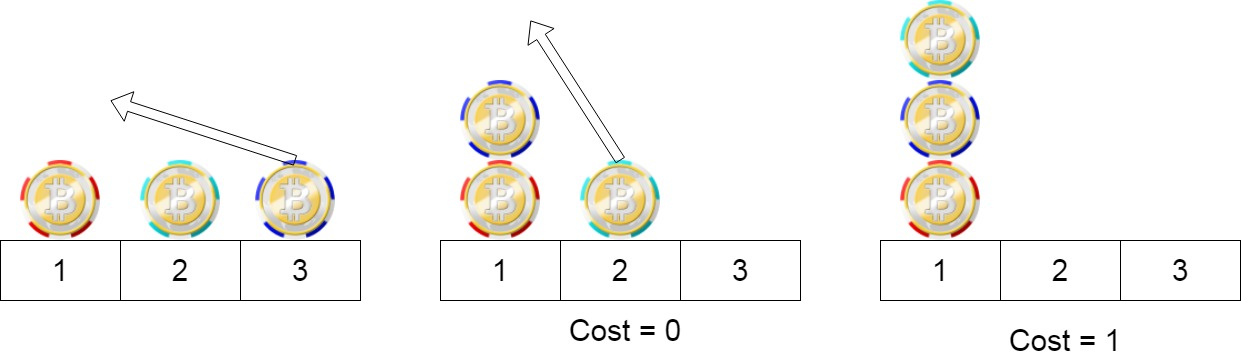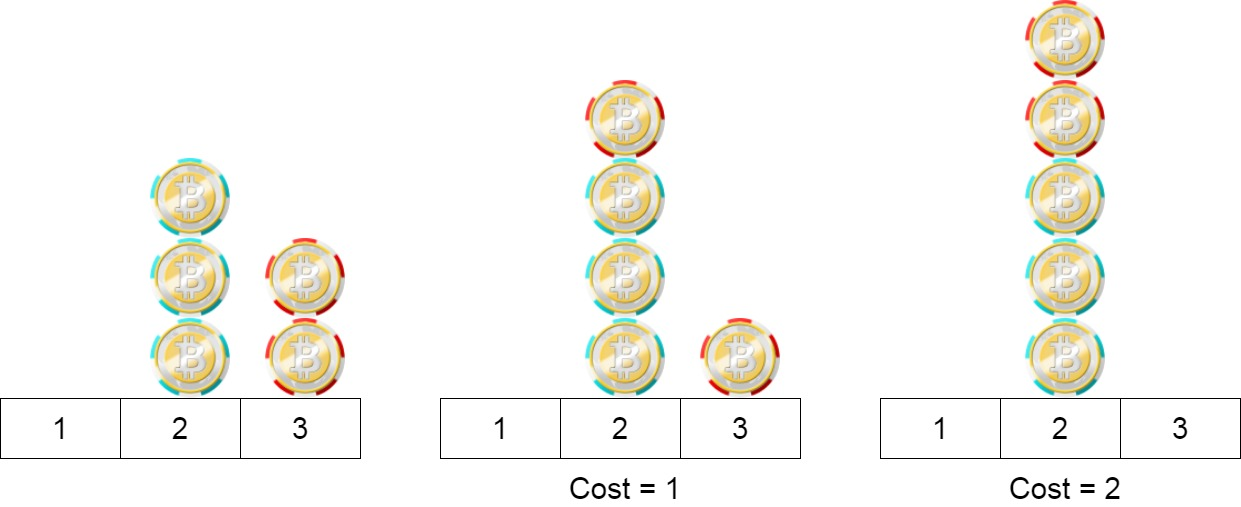## Algorithm

Problem Name: 1217. Minimum Cost to Move Chips to The Same Position

We have `n` chips, where the position of the `ith` chip is `position[i]`.

We need to move all the chips to the same position. In one step, we can change the position of the `ith` chip from `position[i]` to:

• `position[i] + 2` or `position[i] - 2` with `cost = 0`.
• `position[i] + 1` or `position[i] - 1` with `cost = 1`.

Return the minimum cost needed to move all the chips to the same position.

Example 1:```Input: position = [1,2,3]
Output: 1
Explanation: First step: Move the chip at position 3 to position 1 with cost = 0.
Second step: Move the chip at position 2 to position 1 with cost = 1.
Total cost is 1.
```

Example 2:```Input: position = [2,2,2,3,3]
Output: 2
Explanation: We can move the two chips at position  3 to position 2. Each move has cost = 1. The total cost = 2.
```

Example 3:

```Input: position = [1,1000000000]
Output: 1
```

Constraints:

• `1 <= position.length <= 100`
• `1 <= position[i] <= 10^9`

## Code Examples

### #1 Code Example with Java Programming

```Code - Java Programming```

``````
class Solution {
public int minCostToMoveChips(int[] position) {
int evenCount = 0;
int oddCount = 0;
for (int p : position) {
if (p % 2 == 0) {
evenCount++;
} else {
oddCount++;
}
}
return Math.min(evenCount, oddCount);
}
}
``````
Copy The Code &

Input

cmd
position = [2,2,2,3,3]

Output

cmd
2

### #2 Code Example with Javascript Programming

```Code - Javascript Programming```

``````
const minCostToMoveChips = function(position) {
let oddSum = 0, evenSum = 0
for(let i = 0; i < position.length; i++) {
if(position[i] % 2 === 0) evenSum++
else oddSum++
}
return Math.min(oddSum, evenSum)
};
``````
Copy The Code &

Input

cmd
position = [2,2,2,3,3]

Output

cmd
2

### #3 Code Example with Python Programming

```Code - Python Programming```

``````
class Solution:
def minCostToMoveChips(self, chips: List[int]) -> int:
return min(sum((c1 - c2) % 2 for c2 in chips) for c1 in chips)
``````
Copy The Code &

Input

cmd
position = [1,1000000000]

Output

cmd
1

### #4 Code Example with C# Programming

```Code - C# Programming```

``````
using System;

namespace LeetCode
{
public class _1217_PlayWithChips
{
public int MinCostToMoveChips(int[] chips)
{
int odd = 0, even = 0;
foreach (var chip in chips)
{
if (chip % 2 == 0)
even++;
else
odd++;
}

return Math.Min(odd, even);
}
}
}
``````
Copy The Code &

Input

cmd
position = [1,1000000000]

Output

cmd
1